## Sunday, February 26, 2012

### Bézier Curves - Programs for TI nSpire, HP 50g

Introduction

A short definition: a Bézier Curve is a set of parametric equations involving n points:

x(t)=Σ( nCr(n,k) * (1-t)^(n-k) * t^k * x_k) from k=0 to n

y(t)=Σ( nCr(n,k) * (1-t)^(n-k) * t^k * y_k) from k=0 to n

For 0 ≤ t ≤ 1

The curve fits points (x_0, y_0) (when t=0) and (x_n, y_n) (when t=1). The curve approximates the other points.

The degree of the polynomial is n-1.

Bézier curves are used heavily in computer graphics, animation, and font making.

Mike "Pomax" Kamermans provides an awesome primer on Bézier curves.

Béizer Curve Generator - TI nSpire CX
(I am sure this would work with the TI-89 too)

Define LibPub bezier(list)=
Func
© coordinates; Define x (or y)#(t)=bezier(...)
Local n,k
dim(list) - 1 → n
Return Σ( nCr(n,k) * (1-t)^(n-k) * t^k * list[k+1]) from k=0 to n
EndFunc

Save the file, the file name can only be one word. The file must belong to the MyLib folder under the My Documents folder. Please do not forget to refresh the libraries after saving. To refresh, press the doc button, select option 6.

One way to graph a Bézier Curve:

1. Go to, or create a graph screen, or in Scratchpad Graph screen
2. Set to Parametric Mode:

x#(t)=filename\bezier({x coordinates})

y#(t)=filename\bezier({y coordinates})

Set 0 ≤ t ≤ 1

Where # is 1 to 99 and filename is the file that contains the Bezier function.

Bézier Curve for the HP 50g (should work on the 48G and 49G families)

There are two programs in this section. Both are programmed using User RPL.

BEZIER: a curve generator
BEZGRPH: graphs a single Bézier Curve given a list of y coordinates and a list of corresponding x coordinates. BEZIER is required.

Bézier Curve Generator

Level 1: list of coordinates

Program Name: BEZIER
'L' STO
0 'F' STO
'T' PURGE
0 N FOR K
N K COMB
1 'T' - N K - ^ *
'T' K ^ *
L K 1 + GET *
'F' STO+
NEXT F SIMPLIFY FDISTRIB
{N L} PURGE >>

Graphing a Single Bézier Curve:

Level 2: list of y coordinates
Level 1: list of x coordinates

Program Name: BEZGRPH
BEZIER SWAP BEZIER
+ STEQ
PARAMETRIC
{T 0 1} INDEP
AUTO ERASE
DRAW LABEL DRAX PICTURE >>

Notes:
1. The program BEZIER is required.
2. Standard mode (STD) set is used to make labels less obtrusive
3. {T 0 1} INDEP sets T to be the independent variable with the range (0, 1)
Parametric Equations on the HP 50g: XY#(T)=X(T)+i*Y(T)

Example:
Draw a Bézier curve about the points (0,3), (1,2), (0,0), (-1,-1), and (4,-4)
List of y coordinates: {3, 2, 0, -1, -4}
List of x coordinates: {0, 1, 0, -1, 4}

Equations:
x(t) = 4t^4 + 8t^3 - 12t^2 + 4t
y(t) = -5t^4 + 8t^3 - 6t^2 - 4t + 3

TI nSpire CAS CX: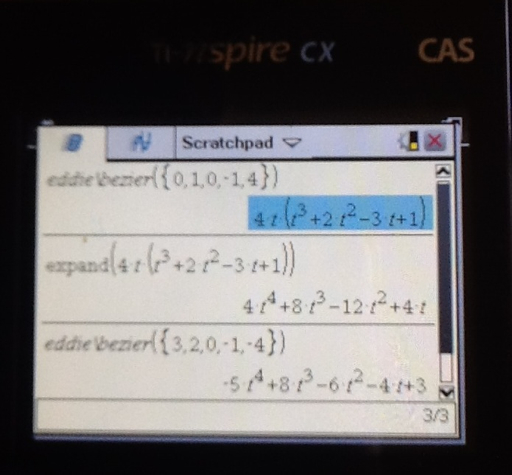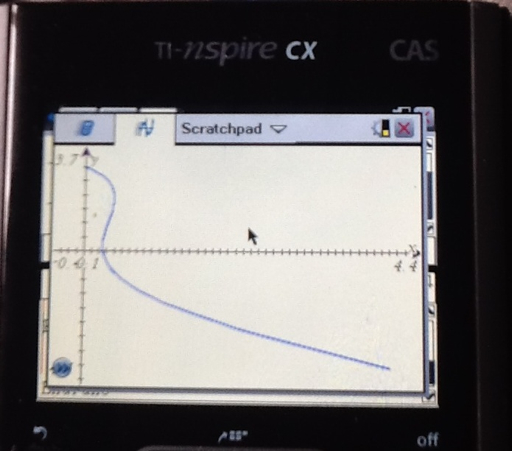HP 50g: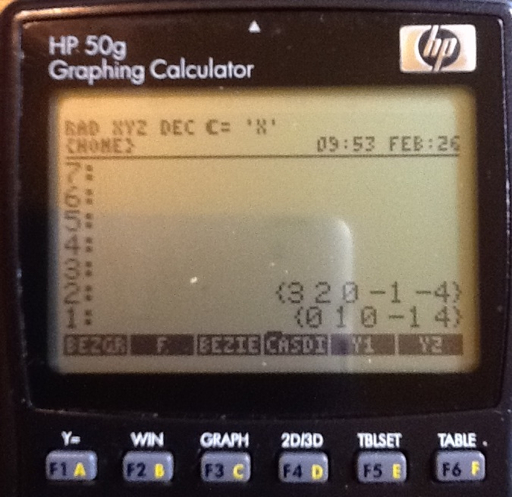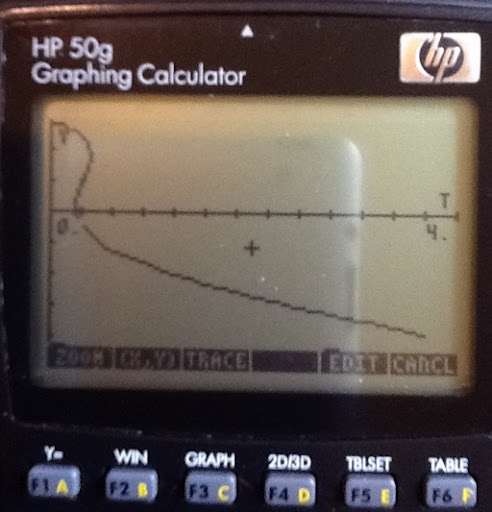Hope you enjoyed this blog. Take care as always, Eddie

This tutorial is property of Edward Shore. © 2012

## Wednesday, February 22, 2012

### An Approach to Plotting Functions in the Complex Plane using Parametric Mode

Graphing functions on the complex plane is not easily handled on a graphing calculator. Few options exist: (1) build a program to build an x-list and a y-list and build a Scatterplot, and (2) use the parametric graphing mode using a numerous amount of paths.

All screen shots are taken with an iPad 2 and a TI nSpire CX CAS calculator.

The complex plane is shown below: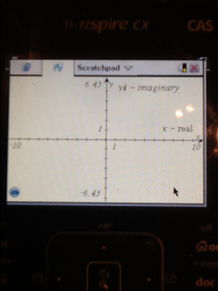There are pros and cons to each method. The pro of method (1) is that you would get a complete picture of any desired mesh. However, you are also faced with high execution time, even on the best graphing calculators, a lot of RAM usage, and you can usually only use a small area to graph on: for example the interval [-2,2] with 25 point intervals.

Method (2) involves parametric graphing that splits the function into two parts: the real component x(t), and its imaginary component y(t). For any complex function f(z):

x(t) = real(f(z))
y(t) = imag(f(z))

You will either have to separate the real and imaginary components, or if you graphing calculator can handle complex operations, use the real and imag functions.

Caution: If you calcualtor can handle complex numbers, some may not have a built in routine for the trigonometric or advanced operations. This applies to the TI-84 family, and most (if not all) Casio graphing calculators. I list some advanced operations for convenience.

Source: HP-41C Math Pac, Hewlett Packard, 1980?

Let z = x + yi, r = abs(z), θ = arg(z) = angle(z), i = √ -1

ln z = ln r + θ i
a ^ z = e ^ (z ln a)
sin z = sin x cosh y + i cos x sinh y
cos z = cos x cosh y - i sin x sinh y
z ^ n = r ^ n * e ^ ( i n θ )
z ^ ( 1/n ) = r ^ ( 1/n ) * e ^ ( i ( θ / n + ( 2 π k ) / n ) ) , k = 0, 1,...,n - 1
e ^ z = e ^ x * cos y + i * e ^ x * sin y

There is one big obstacle with using the parametric mode. There is only one independent variable, t. So using a set of parametric equations describe only one path.

For example, in graphing sin z set the pair of parametric equations as:
x(t) = real(sin(t + t i))
y(t) = imag(sin(t + t i))

graphs sin z along the path y = x. (x=t, y=t)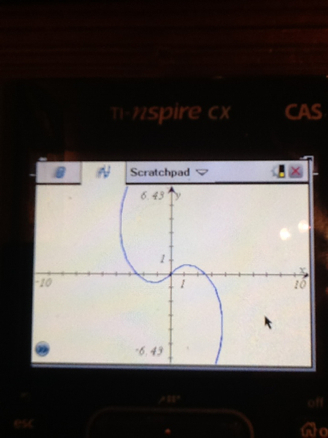In order to get a more complete picture (or really, just more detail), you will have to repeat the pair, but using different paths.

Examples:
For path y = x, use t + t i (sub x = t, y = t)
For path y = -x, use t - t i (sub x = t, y = -t)
For path y = 2x, use 2t + t i (sub x = t, y = 2t)
For path y = e^x, use e^t + t i (sub x = t, y = e^t)
For path y = ln x, use t + e^t * i (sub x = e^t, y = t)
For path y = x^n, use t + t^n * i (sub x = t, y = t^n)
For path y^n = x, use t^n + t * i (sub x = t^n, y = t)

This method is good if you don't want to program, just want to get a quicker picture (method (2) still takes a lot of effort), or you are working with a monochromatic screen (most graphing calculators) where too much detail can just "paint the screen black".

Below are examples of graphs of complex functions from the use of method (2). Keep in mind that these are not complete graphs but enough to get some idea of what is going on.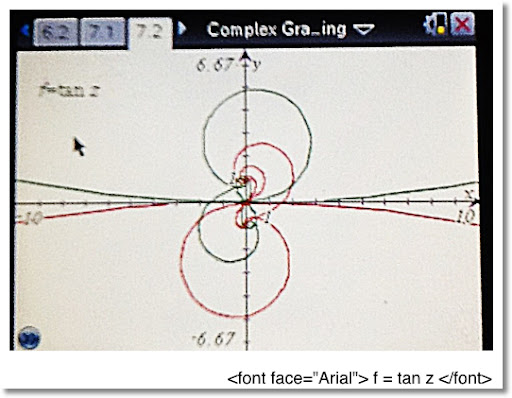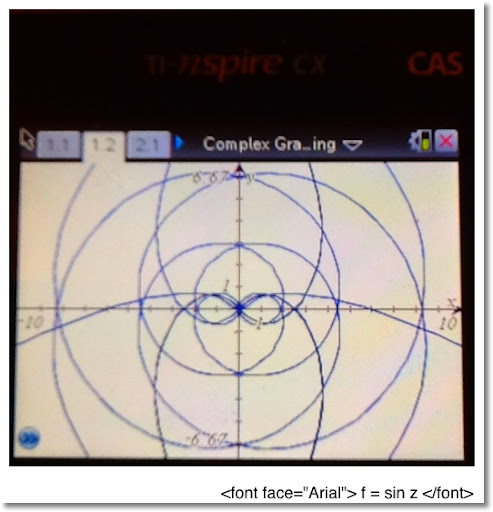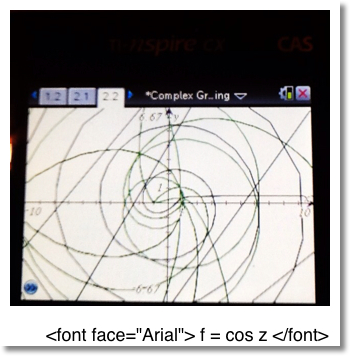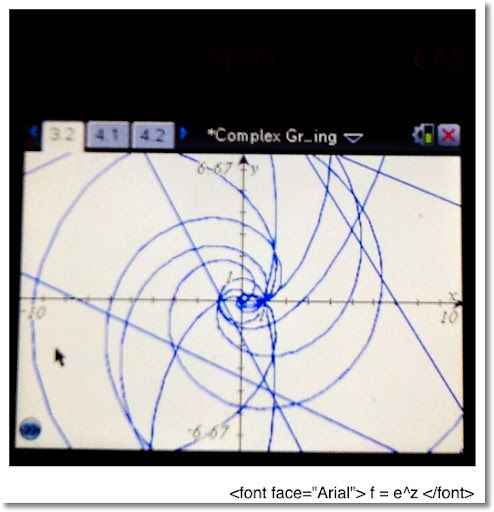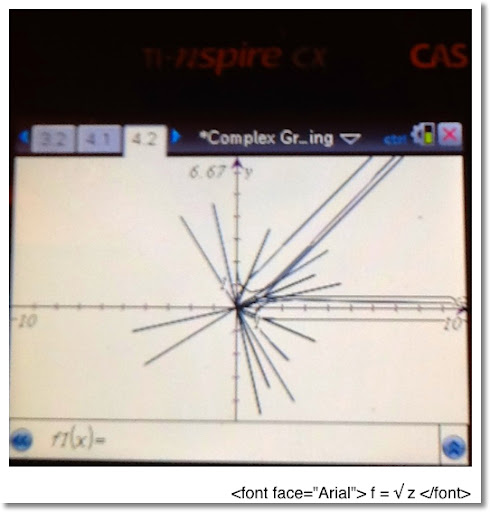Hope you enjoyed this blog. Eddie

This blog is property of Edward Shore. © 2012

## Wednesday, February 8, 2012

### Repeated Applications of Functions

Repeated Functions

If you have ever owned a calculator, chances are you done this: you enter a number and press the square root button many times in a row to see what happens.

For example, you enter say 200. And then you push the square root button. Here are the results for 10 applications of the square root function (to eight decimal places):

14.14213562
3.76060309
1.93922745
1.39256147
1.18006842
1.08630954
1.04226174
1.02091221
1.01040200
1.00518755

Eventually with any positive number, if you press the square root function enough times, you get 1 as a repeated answer.

The following graph shows three functions:

The function in red is the normal square root function.

The function in green shows what happens when the square root function is applied three times to each number.

The function in blue shows what happens when the square root function is applied five times to each number.

Each of the graphs on this blog have been produced using an TI nSpire CAS calculator. All photographs were taken using an iPad 2.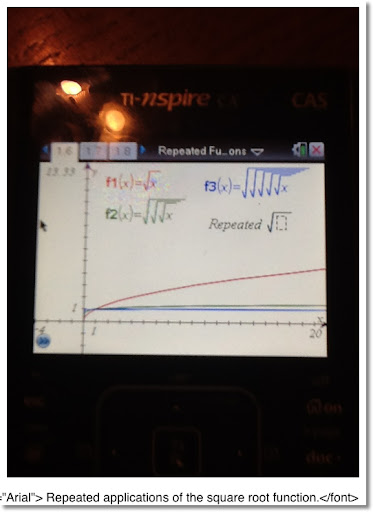Finding a Closed Form for Repeating the Square Root

If we want to describe what a function would look like when applying the square root n times, we can use a nice, closed, and compact formula.

Let f(x) = √ x = x ^(1/2)

Then

f^n(x) = x ^ 1/2 ^ 1/2 ^ .... ^ 1/2 (n times)
= x ^ (1/ (2^n))
= x ^ (2 ^ -n)

Repeated Sine and Logarithm

Now let's apply common scientific functions and repeat them. First the sine function for x ∈ [-3 π, 3 π].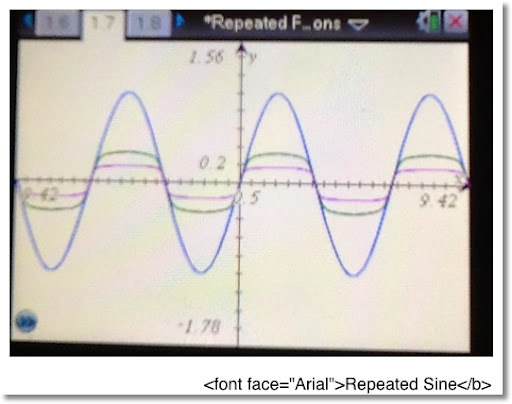The graph in blue represents the normal sine function. (x is in radians)

The graph in green is sine repeated 25 times.

The graph in magenta is sine repeated 100 times.

Note with repeated applications of sine, the graph gets more flatter, towards 0, for each point. Could the approximation formula sin x ≈ x have something to do with this?

This next graph shows what happens when you repeatedly apply the natural logarithmic function: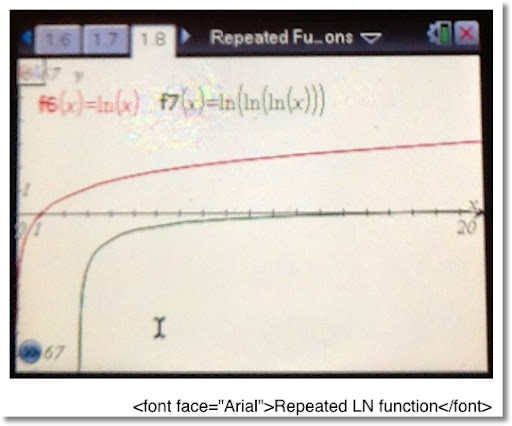The graph in red is the normal natural logarithmic function.

The graph in green represents the natural logarithm function applied three times.

Something interesting happens with repeating the natural logarithm function. Eventually, you will get a complex number (or an error message on a standard scientific calculator).

Cases in point:

When x=2,
ln 2 = 0.69314718 (to eight decimal places)
ln ln 2 = -0.36651292
ln ln ln 2 = -1.00372150 + π i

When x = 50
ln 50 = 3.91202301 (to eight decimal places)
Repeated applications of the natural logarithm yield:
1.36405463
0.31046161
-1.16969502
0.15674305 + π i

I hope you enjoy this blog, until next time,

Eddie

This blog is property of Edward Shore. © 2012

## Monday, February 6, 2012

### What a find: An Atari Calculator

I went to the Pasadena City College Swap Meet yesterday, and I found this calculator.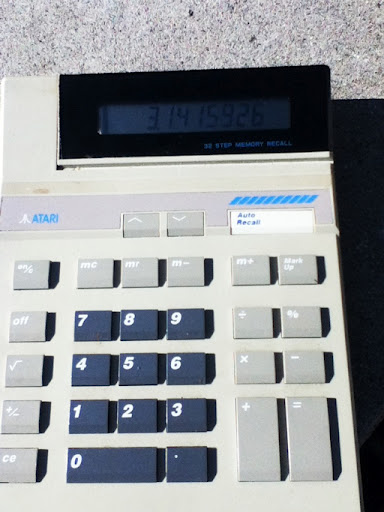Pictured is the Atari CC 1900 calculator. While it isn't programmable, the calculator features a memory of 32 steps. I picked this up for \$5. It looks like this calculator was produced in the 1980s, but I could be wrong on that. Thankfully it runs on two AAA batteries.

This is not the only model of Atari calculators as smaller and solar versions were produced.

Some info about the Atari CC 1900 that I found.

Pasadena City College Flea Market - 1st Sunday of every month

I am looking for a manual.

Take care, Eddie

### What to do next?

I think I want to tackle chaos theory or the study of patterns next.

### HP 32S II Statistical Formulas

HP 32S II Statistical Formulas Statistics Formulas The 32S II can store formulas for evaluations, including formulas involving stat variable...